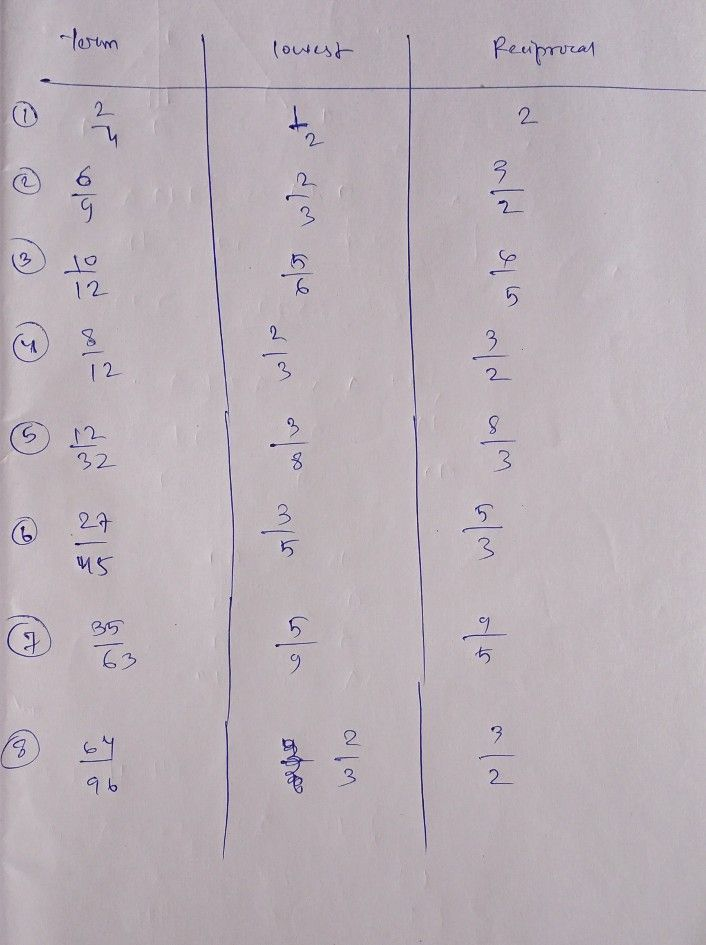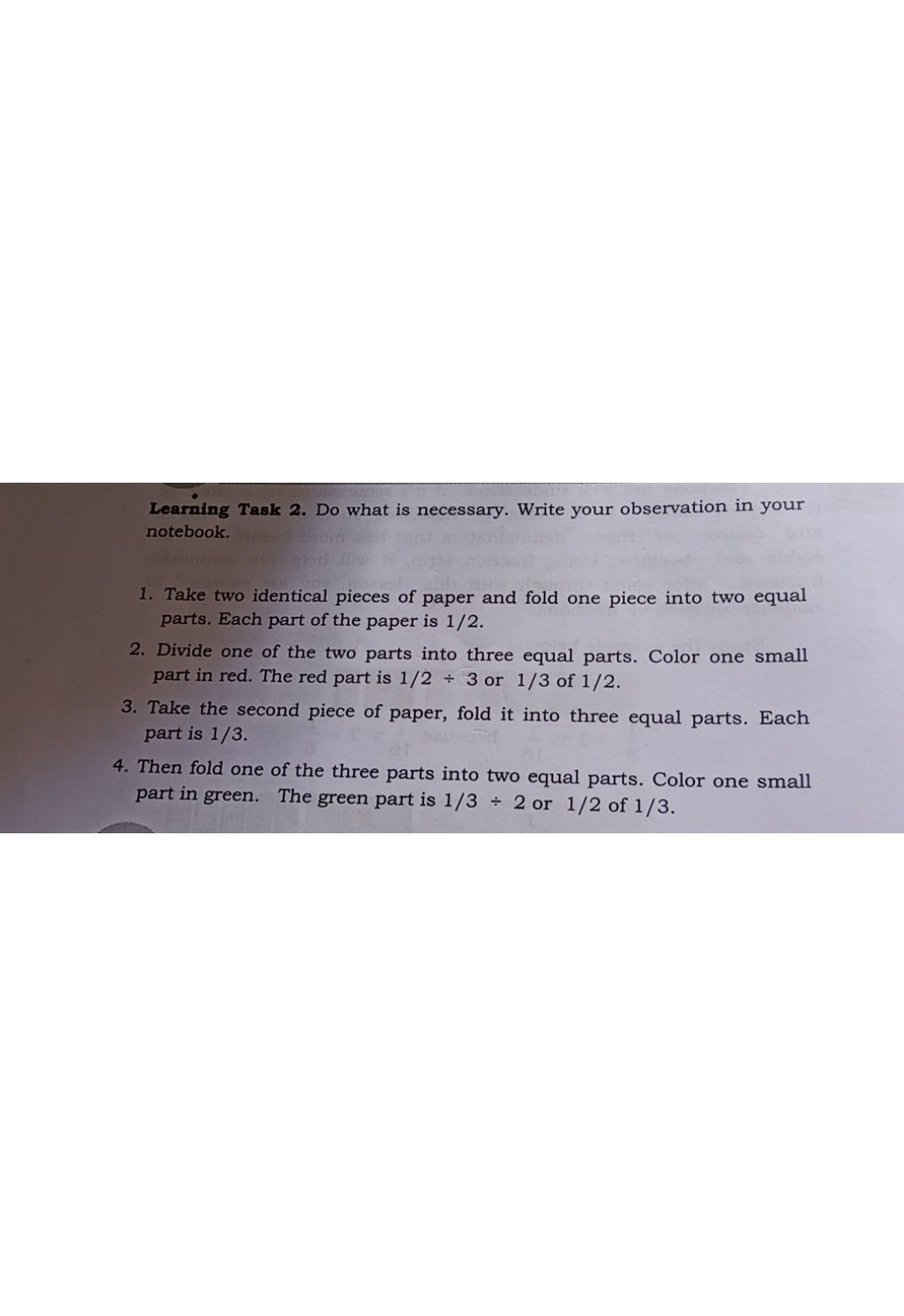Symbol
ProblemLearning Task $1.$ Give the lowest term then write the reciprocal of the following fractions. Write your answer on your notebook. $1\right)$ $\dfrac {2} {4}$ $2\right)$ $\dfrac {6} {9}$ $3\right)$ $\dfrac {10} {12}$ $4\right)$ $\dfrac {8} {12}$ $5\right)$ $\dfrac {12} {32}$ $6\right)$ $\dfrac {27} {45}$ $7\right)$ $\dfrac {35} {63}$ $8\right)$ $\dfrac {64} {96}$
1st-6th grade
Algebra
Search count: 117
SolutionQanda teacher - AnamikaStudentQanda teacher - Anamika
Please ask in another Question. According to Rule of QandA only one solution is allowed for one question. If you have more questions, Visit my PROFILE, Click on ? : ? and upload your question.